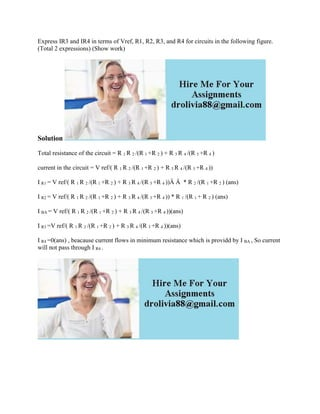Ce diaporama a bien été signalé.
Le téléchargement de votre SlideShare est en cours. ×

# Express IR3 and IR4 in terms of Vref- R1- R2- R3- and R4 for circuits.docx

Publicité
Publicité
Publicité
Publicité
Publicité
Publicité
Publicité
Publicité
Publicité
Publicité
PublicitéChargement dans…3
×

## Consultez-les par la suite

1 sur 1 Publicité

# Express IR3 and IR4 in terms of Vref- R1- R2- R3- and R4 for circuits.docx

Express IR3 and IR4 in terms of Vref, R1, R2, R3, and R4 for circuits in the following figure. (Total 2 expressions) (Show work)
Solution
Total resistance of the circuit = R 1 R 2 /(R 1 +R 2 ) + R 3 R 4 /(R 3 +R 4 )
current in the circuit = V ref/( R 1 R 2 /(R 1 +R 2 ) + R 3 R 4 /(R 3 +R 4 ))
I R1 = V ref/( R 1 R 2 /(R 1 +R 2 ) + R 3 R 4 /(R 3 +R 4 ))Â Â  * R 2 /(R 1 +R 2 ) (ans)
I R2 = V ref/( R 1 R 2 /(R 1 +R 2 ) + R 3 R 4 /(R 3 +R 4 )) * R 1 /(R 1 + R 2 ) (ans)
I BA = V ref/( R 1 R 2 /(R 1 +R 2 ) + R 3 R 4 /(R 3 +R 4 ))(ans)
I R3 =V ref/( R 1 R 2 /(R 1 +R 2 ) + R 3 R 4 /(R 3 +R 4 ))(ans)
I R4 =0(ans) , beacause current flows in minimum resistance which is providd by I BA , So current will not pass through I R4 .
.

Express IR3 and IR4 in terms of Vref, R1, R2, R3, and R4 for circuits in the following figure. (Total 2 expressions) (Show work)
Solution
Total resistance of the circuit = R 1 R 2 /(R 1 +R 2 ) + R 3 R 4 /(R 3 +R 4 )
current in the circuit = V ref/( R 1 R 2 /(R 1 +R 2 ) + R 3 R 4 /(R 3 +R 4 ))
I R1 = V ref/( R 1 R 2 /(R 1 +R 2 ) + R 3 R 4 /(R 3 +R 4 ))Â Â  * R 2 /(R 1 +R 2 ) (ans)
I R2 = V ref/( R 1 R 2 /(R 1 +R 2 ) + R 3 R 4 /(R 3 +R 4 )) * R 1 /(R 1 + R 2 ) (ans)
I BA = V ref/( R 1 R 2 /(R 1 +R 2 ) + R 3 R 4 /(R 3 +R 4 ))(ans)
I R3 =V ref/( R 1 R 2 /(R 1 +R 2 ) + R 3 R 4 /(R 3 +R 4 ))(ans)
I R4 =0(ans) , beacause current flows in minimum resistance which is providd by I BA , So current will not pass through I R4 .
.

Publicité
Publicité

### Express IR3 and IR4 in terms of Vref- R1- R2- R3- and R4 for circuits.docx

1. 1. Express IR3 and IR4 in terms of Vref, R1, R2, R3, and R4 for circuits in the following figure. (Total 2 expressions) (Show work) Solution Total resistance of the circuit = R 1 R 2 /(R 1 +R 2 ) + R 3 R 4 /(R 3 +R 4 ) current in the circuit = V ref/( R 1 R 2 /(R 1 +R 2 ) + R 3 R 4 /(R 3 +R 4 )) I R1 = V ref/( R 1 R 2 /(R 1 +R 2 ) + R 3 R 4 /(R 3 +R 4 ))Â Â * R 2 /(R 1 +R 2 ) (ans) I R2 = V ref/( R 1 R 2 /(R 1 +R 2 ) + R 3 R 4 /(R 3 +R 4 )) * R 1 /(R 1 + R 2 ) (ans) I BA = V ref/( R 1 R 2 /(R 1 +R 2 ) + R 3 R 4 /(R 3 +R 4 ))(ans) I R3 =V ref/( R 1 R 2 /(R 1 +R 2 ) + R 3 R 4 /(R 3 +R 4 ))(ans) I R4 =0(ans) , beacause current flows in minimum resistance which is providd by I BA , So current will not pass through I R4 .